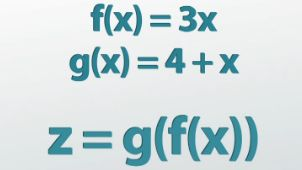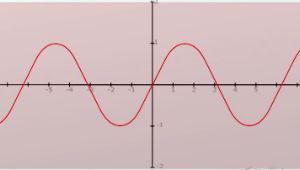# Compounding Functions and Graphing Functions of Functions

Lesson Transcript
Instructor: Robert Egan
We know that functions map numbers to other numbers, so what happens when you have a function of a function? Welcome to functions within functions, the realm of composite functions!

## Functions

Recall that functions are like a black box; they map numbers to other numbers. If y is a function of x, then we write it as y=f(x). And for this function, we have an input, x, and an output, y. So x is our independent variable, and y is our dependent variable. Our input will be anywhere within the domain of the function, and our output will be anywhere within the range of the function. So perhaps it's not too much of a stretch to know that you can combine functions into a big function.

An error occurred trying to load this video.

Try refreshing the page, or contact customer support.

Coming up next: Understanding and Graphing the Inverse Function

### You're on a roll. Keep up the good work!

Replay
Your next lesson will play in 10 seconds
• 0:06 Functions
• 0:58 Composite Functions
• 4:01 Domain and Range of…
• 7:06 Lesson Summary
Save Save

Want to watch this again later?

Log in or sign up to add this lesson to a Custom Course.

Timeline
Autoplay
Autoplay
Speed Speed## Composite Functions

In math, this is known as a composition of functions. Here you start with x, and you use it as input to a function, y=f(x). And you're going to put that as input into a second function, g. So if we have a function y=f(x), and we want to plug it into z=g(y), we can end up with z=g(f(x)). This is a composite function.

When you're looking at composite functions, there are two main points to keep in mind. First, you need to evaluate the function from the inside out. You need to figure out what f(x) is before you figure out what g is. Say we have the function f(x)=3x, and we have another function g(x)= 4 + x. I'm going to find z when x=2. We're going to find f(x) when x=2 for f(2)= 3 * 2, which is 6. Saying g(f(2)) is like saying g(6). We do the same thing and say g(6) = 4 + 6. Well, that's 10, so z is just 10.

The second thing to keep in mind is that g(f(x)) does not equal f(g(x)). There are some cases where it can, but in general, it does not. So if we use f(x)=3x and g(x)=x + 4, then let's look at the case where x=0. Then g(f(0)), where f(0) is 0 * 3 - well that's just zero, so I'm looking at g(0). I plug zero in for x here, and it's just 4. Now, if I look at f(g(0)), that's like saying f(4), and that gives me 12. f(g(0))=12, and g(f(0))=4. Those are not the same. So, g(f(x)) does not equal f(g(x)).## Domain and Range of Composite Functions

What happens to the domain and range of a composite function? Well, if we have the function g(x), we have some domain and some range for g(x). Separately we've got a domain for f(x) and a range for g(x). If I write f(g(x)), then the output of g(x), which is the range, has to be somewhere in the domain of f(x). Otherwise, we could get a number here that f(x) really doesn't know what to do with. What does all this really mean? Consider the function f(x)=sin(x).

To unlock this lesson you must be a Study.com Member.
Create your account

### Register to view this lesson

Are you a student or a teacher?

### Unlock Your Education

#### See for yourself why 30 million people use Study.com

##### Become a Study.com member and start learning now.
Back
What teachers are saying about Study.com
Create an account to start this course today
Used by over 30 million students worldwide